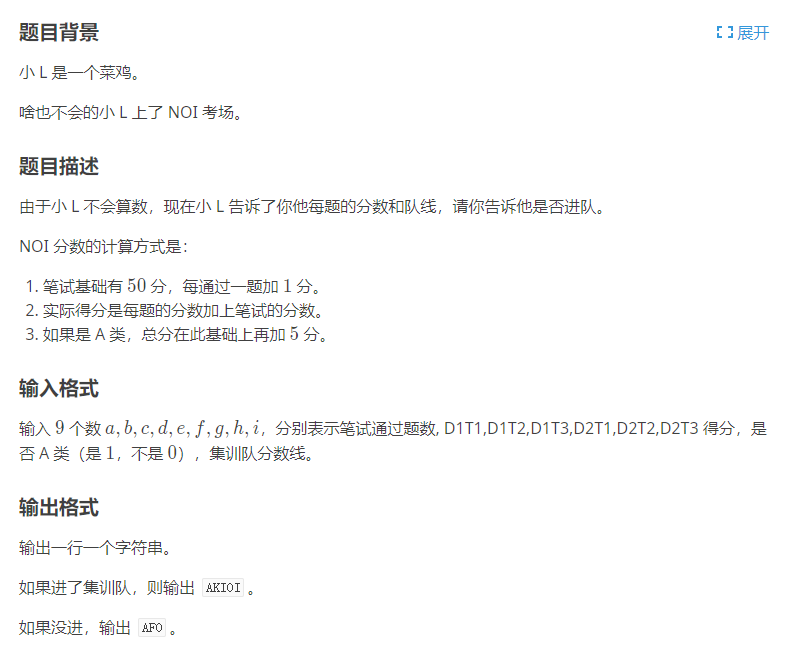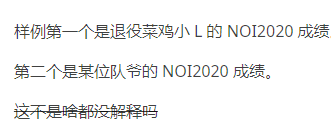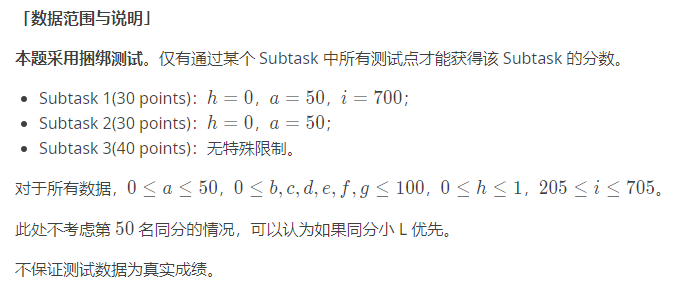• ## NOI

2020-12-07 21:54:02
luogu P6850，NOI，模拟
NOI
题目链接：luogu P6850
题目样例输入1
50 50 72 56 100 40 0 1 446

样例输出1
AFO

样例输入2
50 95 100 64 100 72 30 0 446

样例输出2
AKIOI

样例解释数据范围思路
直接模拟即可。
代码
#include<cstdio>

using namespace std;

int a, b, c, d, e, f, g, h, i;

int main() {
scanf("%d %d %d %d %d %d %d %d %d", &a, &b, &c, &d, &e, &f, &g, &h, &i);

if (50 + a + b + c + d + e + f + g + h * 5 >= i) printf("AKIOI");
else printf("AFO");

return 0;
}

展开全文• NOI 2021基础知识题库 NOI官网发布版.pdfNOIP
• 文章目录前言一、noi linux是什么？二、使用步骤1.虚拟机2.资源总结 前言 noi linux是csp必须用的操作系统 一、noi linux是什么？ noi linux是基于Ubuntu开发的操作系统 二、使用步骤 1.虚拟机 我用的是VMware 16，...
提示：文章写完后，目录可以自动生成，如何生成可参考右边的帮助文档

文章目录
前言一、noi linux是什么？二、使用步骤1.虚拟机2.资源
总结

前言
noi linux是csp必须用的操作系统
一、noi linux是什么？
noi linux是基于Ubuntu开发的操作系统
二、使用步骤
1.虚拟机
我用的是VMware 16，但只适用于win10，而win7则需用VMware 11。
2.资源
VMware 16
许可证：ZF3R0-FHED2-M80TY-8QYGC-NPKYF
YF390-0HF8P-M81RQ-2DXQE-M2UT6
ZF71R-DMX85-08DQY-8YMNC-PPHV8
VMware 11
许可证：1F04Z-6D111-7Z029-AV0Q4-3AEH8
总结
以上是虚拟机推荐
展开全文ubuntu
• 某中学 NOI 教练总结的，发给我学习，分享给大家，总结的还算是可以的。
• 绝对正版的noi2018笔试题库，适合参加noi的dalao们复习用11111111111111111111
• NOI系列比赛平台NOI Linux简介 ppt 2010
• CCF NOI 2021 统一省选（含有 A、B 卷题目）
• NOI 2005年-2009年试题。 第二十二届至第二十六届全国信息学奥林匹克竞赛（NOI）试题。
• 第十九届全国信息学奥林匹克竞赛 PDF版 试题。 NOI2002 PDF版 试题。 含NOI2002第一试、第二试。
• [NOI2010] [NOI2010]海拔 高度只需要0/1，所以一个合法方案就是一个割，平面图求最小割。 [NOI2010]航空管制 反序拓扑排序，每次取出第一类限制最大的放置，这样做答案不会更劣。 考虑如何求每一个的最早时间，同...

[NOI2010]
[NOI2010]海拔
高度只需要0/1，所以一个合法方案就是一个割，平面图求最小割。
[NOI2010]航空管制
反序拓扑排序，每次取出第一类限制最大的放置，这样做答案不会更劣。 考虑如何求每一个的最早时间，同上述拓扑排序，该元素不入队，当无点可拓展时就是最早时间。
[NOI2010]超级钢琴
对每一个左端点维护当前取出了前几大的右端点，用堆+主席树维护。
[NOI2011]
[NOI2011]兔农
不难发现数列模意义下的进程是：斐波那切数列，首项回归0(减一操作)，斐波那切数列...... 也就是说每一段中分别是一个斐波那切数列，故可以分段矩乘。 而模$$K$$意义下斐波那切数列循环节不超过$$6K$$，所以处理出循环节后暴力。
[NOI2011]Noi嘉年华
区间离散化，设$$w_{l,r}$$为区间$$[l,r]$$权值，设$$f_{i,j}$$表示前$$i$$个点场地一答案为$$j$$，场地二的最大答案。 考虑算第二问的答案，对于询问区间，枚举包含它的大区间。类似$$f$$先处理好后缀答案$$g$$。 预处理大区间答案：$$ans_{l,r}=max_{x,y}\{min(w_{l,r}+f_{l-1,x}+g_{r+1,y}\ ,\ x+y)\}$$。 可以发现$$x$$上升$$y$$一定不降(为了平衡两边权值)，所以只用枚举$$x$$，$$y$$用一个指针维护，复杂度$$O(n^3)$$。
[NOI2011]阿狸的打字机
建$$AC$$自动机，相当于询问一个点跳$$fail$$能跳到一条$$dfs$$链上的几个点。 离线$$dfs$$，然后用树状数组实现子树加计算答案。
[NOI2011]兔兔与蛋蛋游戏
把网格图转为二分图博弈，需要支持移动一步维持匹配、判定匹配必须点，找交替路即可。
[NOI2012]
[NOI2012]美食节
暴力，每道菜建点$$v\to T$$，每个厨师建点$$S\to u$$。 对每个厨师，按天数分层建点，第$$i$$层表示做倒数第$$i$$道菜，并把该点向每道菜连边，跑费用流。 可以动态加边，当一个厨师被增广后，再给他建新菜的边，复杂度$$O(nm\sum_{j=1}^p j)$$不满，能过。
[NOI2012]魔幻棋盘
扩欧差分后矩阵修改变成单点修改，拿线段树套线段树分四个象限维护。
[NOI2012]骑行川藏
套拉格朗日最小乘子法公式：$$F(x_{1\sim n},\delta)=f(x_{1\sim n})+\delta g(x_{1\sim n})$$。 那么问题变为求一组解$$(x_{1\sim n},\delta)$$满足每个变量的偏导都是$$0$$，$$x_i$$显然是很好求的，二分即可。 不难发现$$\delta$$与$$F'(x_{1\sim n},\delta)=g(x_{1\sim n})$$呈反比关系，所以乘子$$\delta$$也可以二分求解。
[NOI2012]迷失游乐园
对于一棵树，从根出发最后一定是到一个叶子。当经过一个度数为$$p$$的点时，概率乘上$$\frac{1}{p}$$或$$\frac{1}{p-1}$$。 考虑每条边的贡献，换根$$dp$$即可。基环树由于环很小所以没什么区别，大力讨论起终点的位置然后暴力。
[NOI2013]
[NOI2013]向量内积
目前最喜欢的一道$$NOI$$题。 先考虑$$k=2$$，显然可以构造两个矩阵$$A,B$$，满足$$[c_{i,j}]C=A\times B$$为$$vec_i,vec_j$$的向量内集。 我们现在的目标是判断$$A\times B$$是否为除对角线外全$$1$$的矩阵。 随机化，通过$$rand$$几个行向量与原矩阵向量相乘优化矩乘复杂度，然后判断结果是否相同。 关于$$k=3$$，把矩阵$$C$$的每一项平方即可变为$$k=2$$的问题。 发现$$c_{i,j}=(\sum_{k=1}^n a_{i,k}b_{k,j})^2=\sum_{k=1}^n\sum_{t=1}^n(a_{i,k}a_{i,t})(b_{k,j}b_{t,j})$$，所以扩域即可。
[NOI2013]快餐店
考虑计算快餐店落在每条边上的答案，变为求基环树上到某条边的最长路径，随便$$dp$$一下。
[NOI2013]树的计数
问题变为$$bfn$$序期望分多少层，考虑在一棵树上$$dfs$$的过程，不难发现： 若$$dfn_{bfs_{i}}>dfn_{bfs_{i+1}}$$，则$$i,i+1$$必须分一层，贡献为$$1$$。 若$$bfn_{dfs_{i}}<bfn_{dfs_{i+1}}$$，则$$bfn_{dfs_{i}},bfn_{dfs_{i+1}}$$最多分一层。剩下的未定点贡献均为$$0.5$$。
[NOI2014]
[NOI2014]购票
可以写出一个斜率优化的式子，然后上有根树点分治就行了。
[NOI2014]动物园
建$$next$$树，然后$$dfs$$一遍用栈维护即可。也可以先求$$next$$，然后类似求$$next$$的把答案求出来。
[NOI2015]
[NOI2015]品酒大会
建后缀数组，按照$$Height$$从大到小合并，带权并查集维护答案。
[NOI2015]寿司晚宴
按照$$>\sqrt{n}$$的质因子分层状压$$dp$$。
[NOI2015]小园丁与老司机
暴力$$dp$$，然后把所有路径抠出来后变为$$DAG$$覆盖问题，跑上下界网络流。
[NOI2016]
[NOI2016]区间
枚举$$r$$，$$l$$显然随着$$r$$的增长单调递增。双指针后用线段树判合法，实时更新答案。
[NOI2016]循环之美
纯循环小数满足：$$x(K^t-1)\equiv y(mod K)$$，由于$$x\perp y$$故$$K^t\equiv 1(mod\ y)$$,有解时$$K\perp y$$。 所以$$Ans=\sum_{x=1}^n\sum_{y=1}^m [x\perp y][K\perp y]=\sum_{y=1}^m[K\perp y]\sum_{d|x,d|y}\mu(d)$$。 故$$Ans=\sum_{d}\mu(d)\lfloor \frac{n}{d}\rfloor\sum_{y=1}^{\lfloor \frac{m}{d} \rfloor} [yd\perp K]=\sum_{d}\lfloor \frac{n}{d}\rfloor(\sum_{y=1}^{\lfloor \frac{m}{d} \rfloor}[K\perp y])\mu(d)[d\perp K]$$。 令$$f(d)=\mu(d)[d\perp K]$$，问题变为求$$\sum_{i=1}^n f(d)$$。 令$$g(d)=[d\perp K]$$，可以发现$$f(d)*g(d)=e$$，然后上杜教筛就行了。
[NOI2016]优秀的拆分
使用$$SA$$的顶标法解决。
[NOI2016]国王饮水记
把水位低于首都水位的地方先扔掉，把城市按水位升序排序。然后疯狂找(cai)性(jie)质(lun)。 (1)一个水池只会被合并一次，显然。 (2)最终合并的水池一定是一段后缀且分成若干连续段合并，反证法显然。 (3)把水池分开合并比一起合并更优，感性理解手玩一下的确是这样的。 设$$f_{i,j}$$表示前$$i$$个水池合并$$j$$次的最优解，$$f_{i,j}=min_k\frac{s_i+(f_{k,j-1}-s_k)}{i-k+1}$$，其中$$s_i$$为水位前缀和，左式显然斜率优化。 (4)斜率优化的决策单调，即$$k$$随着$$i$$的增加而不降。利用$$s_{i+1}\ge s_{i}+i$$，带入暴力验证即可。 (5)合并水池长度单调不升，若两段$$l_i <l_{i+1}$$，把$$l_{i+1}$$的第一个元素移到$$l_i$$会更优，列式子暴力验证即可。 (6)长度大于$$1$$的段不会很多，不超过$$log(\frac{nh}{min(h_i-h_j)})$$，即不超过$$14$$，打表可得。 然后利用上述性质，我们只用求解$$f_{i,j\leq 14}$$，最后$$dfs$$一遍，用高精小数类求出精确答案。
[NOI2016]旷野大计算
造计算机神题，题解令开一份：戳这里
[NOI2017]
[NOI2017]整数
先考虑$$|a|=1,b=1$$的暴力，线段树模拟二进制，加法就是向高维找$$0$$，减法就是向高维找$$1$$。 可以发现$$b>1$$其实并没有什么不同，压位即可。
[NOI2017]蚯蚓排队
用链表模拟，每次$$O(K^2)$$暴力合并/删除即可。考虑每个点的贡献可以分析出复杂度为$$O(nK)$$。
[NOI2017]泳池
先把问题转为求面积$$\leq K$$的方案数。预处理长度为$$i(i\leq K)$$的合法方案数，剩下的就是线性递推。 考虑最低点，设$$f_{i,j}$$表示长度为$$i$$，最低点高度为$$j$$的合法方案数，其中$$ij\leq K$$故状态数调和级数。 转移枚举最低点在哪(强制选最小最低点)：$$f_{i,j}=q^j(1-q)\sum_{k=1}^j (\sum_{l=k+1}^{1000}f_{k-1,l})(\sum_{r=k}^{1000}f_{i-k,r})$$。
[NOI2017]游戏
搜一下未确定点的选择，然后上$$2-sat$$。
[NOI2017]蔬菜
首先第$$i-1$$天的蔬菜集合一定是第$$i$$天的子集，所以实际上只用求最大的那天的结果。 建网络流模型，$$S\to$$每种蔬菜，每种蔬菜按时间建一条链，链的容量限制按变质递减，链上每个结点向对应那天连边。 考虑每次增广，可以发现反悔边没有鸟用(每种蔬菜只用一个增广方向)，所以考虑模拟费用流。 不难发现找最长路等价于每次取出价值最大的蔬菜增广，拿个堆维护即可。 最后的问题在于链上递减的容量限制。 因为每种蔬菜对每一天的贡献都是一样的，所以贪心的先增广较晚的天就行了，用并查集搞下。
[NOI2018]
[NOI2018]归程
$$Dij$$预处理$$1$$到每个点的最短路。建$$Kruskal$$重构树，问题变为子树最小值，随便维护下。
[NOI2018]冒泡排序
合法的充要条件为：不存在长度为$$3$$的下降序列。 稍加思考可以得到$$dp$$：设$$f_{i,j}$$表示前$$i$$个的最大值为$$j$$，转移$$f_{i,j}=\sum_{k=1}^j f_{i-1,k}$$，其中$$j\ge i$$。 加了字典序的限制，考虑数位$$dp$$，即我们需要直到一个后缀的方案数。 设$$g_{i,j}$$表示还有$$i$$个数要放，前面放的数的最大值为$$j$$的方案数，转移$$g_{i,j}=\sum_{k=j}^nf_{i+1,k}$$，其中$$j\ge n-i+1$$。 用网格图优化一下$$g$$的求解，然后用树状数组维护合法性，从前往后数位$$dp$$求出答案。
[NOI2018]你的名字
先求$$T$$有多少不同子串，然后求$$T$$的每个前缀在$$S$$上的匹配长度，这个放在$$S$$的$$SAM$$上跑一下即可。 为了防止算重，对$$T$$也建$$SAM$$，然后在这个$$SAM$$上跑一遍把答案跑出来。
[NOI2018]屠龙勇士
用$$set$$把每次战斗的剑搞出来，用扩欧把式子化为同余方程，然后上$$EXCRT$$。
[NOI2018]情报中心
设$$Len_{(u,v)}$$表示路径$$(u,v)$$的长度。根据部分分的提示，分$$S_1,S_2$$两种情况讨论： (1)两条路径的$$lca$$不同： 我们可以把路径$$(u,v)$$拆成两条直上直下的路径$$(u,z)$$，其中$$dep_u>dep_z$$。 那么路径的交是一条从上往下的链，设为$$(a,b)$$，其中$$dep_a>dep_b$$。 考虑枚举$$a$$，那么就是选两条路径$$(u_1,z_1),(u_2,z_2)$$满足$$u_1,u_2$$在$$a$$的不同子树中。 这组选择的答案为：$$Len_{(u_1,v_1)}+Len_{(u_2,v_2)}+val_1+val_2-dis_a+max(dis_{z_1},dis_{z_2})$$。 设$$f_{x,j}$$表示$$u$$在$$x$$子树内，$$dep_z=j$$的最优信息，用线段树合并维护一下这个值并求答案。 (2)两条路径的$$lca$$相同： 首先对每个$$lca$$建虚树。对每个虚树分别求一遍。 设$$(u_1,v_1),(u_2,v_2)$$的路径交为$$(a,b)$$，其中$$u_1,u_2$$在$$a$$子树中。 一个关键的转化：答案的两倍=$$Len_{(u_1,v_1)}+Len_{(u_2,v_2)}+val_1+val_2+dis(u_1,u_2)+dis(v_1,v_2)$$。 考虑枚举$$a$$，那么令$$W_{(u_1,v_1)}=Len_{u_1,v_1}+val_1+dis_{u_1}$$。 答案变为$$dis(v_1,v_2)+W_{(u_1,v_1)}+W(u_2,v_2)-2dis_{a}$$，$$-2dis_a$$扔掉，就是一个集合直径问题。 额外挂的边权值可能为负，但分析可知这张图依旧满足正权直径定理，所以暴力合并即可。 结合两部分就是正解，第一部分为$$O(nlogn)$$，第二部分为$$O(n+m)$$，需要注意实现细节。

转载于:https://www.cnblogs.com/GuessYCB/p/10753622.html
展开全文• NOI2015试题，有2天的题= = PDF格式的，压缩了一下
• NOI2021游记 前言 OI生涯中最后一次NOI了，既有些伤感，又有些期待，愿能为我的OI生涯画上不留遗憾的一笔。 Day -？ 临近NOI，也许别人会感到紧张和焦虑，但我却几乎没有这种情绪，也许是已经释怀了吧，多年的...
NOI2021游记
前言
OI生涯中最后一次NOI了，既有些伤感，又有些期待，愿能为我的OI生涯画上不留遗憾的一笔。
Day -？
临近NOI，也许别人会感到紧张和焦虑，但我却几乎没有这种情绪，也许是已经释怀了吧，多年的拼搏和努力汇聚到一起，最后的一站需要更多的机遇。保持了一个平常心，以我的水平退役了也在情理之中，所以就不想那么多了。
Day -1
提前来到了余姚梦麟中学，宿舍环境还不错，不过由于提前来到学校，饭堂还没有正式开，所以吃了一天的土。  这两天余姚刚好有台风，所以开幕式也取消了，是摸鱼的好时机！  摸了一天。
Day 0
摸了一天（身边的人都在卷）。由于一直在宿舍里，加上学校在地势很高的地方，台风的影响并不大。
Day 1
回想起去年，我在NOI赛场上折戟沉沙，今年，我又回来了，心中掀起一点浪花，但很快又平静了下来。  9点开始，在大体育场内感受不到外面风雨交加，只是监考人员提到因为台风原因可能会断电而有点小担忧。  比赛开始了。  一开始我的状态很不好，思路转得也很慢，第一题看一眼就以为会了，结果再看才发现看错了题。又仔细想了想感觉可以用树剖搞，记录了每一个点清空的时间来维护轻边的结果，再单独用线段树维护重边的结果，想了一个小时才理清思路，打了四十分钟搞定了，测了随机数据需要0.9s（并没有很慌，虽然赛后发现满二叉树要更慢，但是出题人不会放三个满二叉树来卡常吧）。  然后看了看T2，看完题意就会75pts了，感觉已经有很多分了，想了一会儿也不会100pts，就去搞T3了。  我并没有直接会正解，而是抱着打满部分分的心态去做这题的（毕竟NOIT3在我的了解中都是我切不掉的，并且感觉这题是分类讨论，所以就分开打了）。先搞了个

n

2

n^2

，然后想了一下搞了个建树，又讨论了一下搞了

k

=

0

/

1

k=0/1

。然后重新想

k

=

2

k=2

的情况，大概在最后1h的时候想到不用讨论，直接建虚树就好了，0.5h冲了一个暴力的虚树，再把之前的

n

2

n^2

拼过来，运气还不错，几乎没有怎么调就过了。  最后0.5h我拍了一下T3，检查了一下所有的题目，并没有去仔细地想T2，感觉最后的时间太紧了可能冲不了100pts。  评测的结果是

100

+

75

+

100

100+75+100

，T1、T3差点被卡常，大样例还是非常不错的，没有让我fst。  出来感觉自己分数还蛮高，结果一问才发现T2也是sb题，一堆人切了，结果一堆人AK了（Day1还是太水了吗？）。之后打听了一下有30多个人AK了，感觉以我的分数40多名应该有吧。  同校的其它同学不少切掉了T2，但是没有搞出来T3，分数比我低一点。  然后就摸啊摸。
Day 1.5
嘉年华，gu了，7个人在宿舍里面激情generals了一天，被打爆了Orz。
Day 2
今天的题目也许会很难（奶一口）。八点准时开始。看了题目，第三题题意很复杂，但是没有深究就先取搞第一题了。T1一看是随机，可能是乱搞。再想了一下，这种二进制匹配的题目不能用trie，一般都要分块，随便分了个块，感觉要在某个块内重合尽量多，再看了看

k

≤

15

k\le15

，发现如果分16个块那么必定有一个是完全一样的，大概在1.5h的时候搞定了T1。T2感觉可以维护一个矩阵，连续的

W

W

和

E

E

都可以写成矩阵，用splay维护即可做那些操作。只不过中途换了个做法，再加上我将相邻的

W

,

E

W,E

缩到一起做，所以很麻烦，没有搞出来。最后1h的时候很紧张，把T2的正解放弃了，补了部分分50pts，最后15min打了T3的12pts。感觉凉了，T2应该是裸题，但是我并没有实现出来，T3有很多部分分我也没有拿满。。。出来之后发现zfy和gmh都切了T2，T1并没有我想象中的那么多人切。最后得分

100

+

45

+

12

100+45+12

，T2挂了5pts。之后也没有什么事情干了，感觉退役了，Day1分差都不大，Day2T2不难我没有搞出来。最后是

100

+

275

+

157

=

532

100+275+157=532

。之后突然得知进队了的消息，一开始难以置信，直到最后的总榜出来时候才如释重负，队线比我想象的要低，可能是Day2T2切的人也不到50个吧。
小结
总体而言这次的NOI难度偏低，进队容易，Day1很简单，并且有4道模板题（Day1 T1,2,3,Day2 T2），感觉今年就是Day1打满暴力，Day2切掉T1就能进队了吧。  Day1T2猜一下结论应该可以发现中间的不经过重复点没有意义吧，Day2T2能够转化为更简单的形式，难怪我的方法打不出来。  Day1发挥的还算可以，Day2及时补部分分的策略还算明智，这两天的调试也不多，可见锻炼代码实现能力的重要性了。  OI就是这样，有人欢喜有人忧，gmh和597都差点进队了，只能说是运气和实力一样重要吧，之后也是一个新的开始了，愿我们前程似锦、一路光明！ 
展开全文• NOI WC 2007的比赛试题，第一题已经绝版，各大OJ均无，可自行下载
• 全国信息学奥林匹克竞赛的2015年冬令营课件（NOI WC2015，不包含当年题目），对oi选手帮助很大
• AFO后发福利，noi模拟题（有代码，有解题报告），可对拍
• AFO后发福利，noi模拟题（有代码，有解题报告），可对拍
• 全国信息学奥林匹克竞赛的2014年冬令营课件（NOI WC2014，不包含当年题目），对oi选手帮助很大...